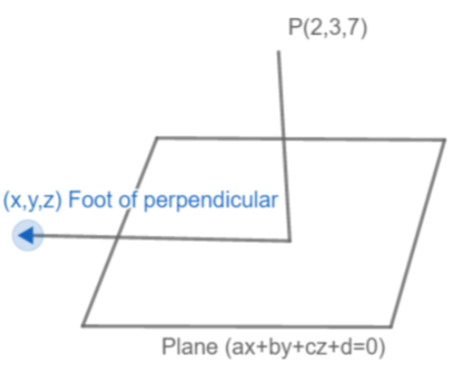QuestionAnswers

# Find the coordinates of the foot of the perpendicular drawn from (2, 3, 7) from the plane 3x-y-z = 7, also find the length of $\left| \dfrac{ax+by+c-d}{\sqrt{{{a}^{2}}+{{b}^{2}}+{{c}^{2}}}} \right|$

Hint: From the plane equation find the foot of the perpendicular which lies in the plane and then, find the length of perpendicular as the distance any point from plane. Use the formula $\dfrac{x-h}{a}=\dfrac{y-k}{b}=\dfrac{z-l}{c}=\text{any constant say }\!\!'\!\!\text{ r }\!\!'\!\!\text{ }$ for finding the equation of plane, where (h, k, l) represent coordinates of point (2, 3, 7) and a, b, c, d are the coefficients of plane ax+by+cz+d=0, i.e 3x-y-z=7 in our case. We will use $\text{Length=}\left| \dfrac{ax+by+c-d}{\sqrt{{{a}^{2}}+{{b}^{2}}+{{c}^{2}}}} \right|$ to find the length of the perpendicular.

Complete step-by-step solution:
First, we must use the formula when a perpendicular is drawn from a point to the plane. To find out the foot of perpendicular, suppose, the point P (h, k, l) and the plane is ax + by + cz + d=0, then the equation of plane:
$\dfrac{x-h}{a}=\dfrac{y-k}{b}=\dfrac{z-l}{c}=\text{any constant say }\!\!'\!\!\text{ r }\!\!'\!\!\text{ }$
Here, in the question, we have been given the point as P (2, 3, 7) and the plane as 3x-y-z-7=0.
When we compare with general form, then we get a = 3, b = -1, c = -1, d = -7 and h = 2, k = 3, l = 7.
Therefore, substituting values, we get
$\dfrac{x-2}{3}=\dfrac{y-3}{-1}=\dfrac{z-7}{-1}=r$
x, y, z in the above equation are the coordinate of the foot of the perpendicular. Now, converting these coordinates in terms of r, we get
\begin{align} & \dfrac{x-2}{3}=r\Rightarrow x=3r+2 \\ & \dfrac{y-3}{-1}=r\Rightarrow y=3-r \\ & \dfrac{z-7}{-1}=r\Rightarrow z=7-r \\ \end{align}
(x, y, z) is the foot of perpendicular, which lies in the plane.
So, we get that (x, y, z) must satisfy the plane equation i.e.
$\text{3x}-\text{y}-\text{z}-\text{7}=0$Putting the values of $\left( \text{x},\text{ y},\text{ z} \right)\to \left( 3r+2,3-r,7-r \right)$ in the above equation, we get
\begin{align} & \Rightarrow 3\left( 3r+2 \right)-\left( 3-r \right)-\left( 7-r \right)-7=0 \\ & \Rightarrow 9r+6+r-3+r-7-7=0 \\ & \Rightarrow 11r=11 \\ & \Rightarrow r=1 \\ \end{align}
Therefore, the coordinates of the foot becomes,
$\left( 3r+2,3-r,7-r \right)=\left( 5,2,6 \right)$
Now, let’s find the length of perpendicular from P onto the given plane:
$\text{Length=}\left| \dfrac{ax+by+c-d}{\sqrt{{{a}^{2}}+{{b}^{2}}+{{c}^{2}}}} \right|$
We have the point as P (2, 3, 7) and plane as 3x-y-z-7=0, so we can substitute in the above formula and we get
\begin{align} & \text{Length of perpendicular=}\left| \dfrac{3\left( 2 \right)-1\left( 3 \right)-1\left( 7 \right)-7}{\sqrt{{{3}^{2}}+{{\left( -1 \right)}^{2}}+{{\left( -1 \right)}^{2}}}} \right| \\ & \Rightarrow \dfrac{\left| -11 \right|}{\sqrt{11}}=\dfrac{11}{\sqrt{11}}=\sqrt{11}\text{ units} \\ \end{align}

Note: $\left| {} \right|$ is the sign of modulus. Modulus always takes the positive values of numbers for example:
$\left| -2 \right|=2\text{ and }\left| 2 \right|=2$
Then, $\left| -2 \right|=\left| 2 \right|=2,$ both will have same values.
In the above solution, we have used two formulas and it is very important that students remember them to solve and get the right answers. Students can easily remember them using certain tricks. Consider the point is P (h, k, l) and the plane is ax + by + cz + d=0. Now to find the equation of a plane, students have to consider the equation of a plane, take variables and then deduct the coordinates of a point from it and finally divide the coefficients of the plane by this difference. Doing this for each variable and equating to r, they will get the required equation.